# A teacher

A teacher baked 5 dozen cookies for the 16 students in her class. If the teacher divided the cookies equally among her students, how many cookies did she give each student?

x =  3.75

### Step-by-step explanation: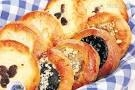Did you find an error or inaccuracy? Feel free to write us. Thank you!Tips to related online calculators
Need help to calculate sum, simplify or multiply fractions? Try our fraction calculator.
Do you want to perform natural numbers division - find the quotient and remainder?

## Related math problems and questions: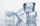Miriam is making 3 1/2 dozen cookies. If 2/7 of the cookies have icing, how many dozen cookies have icing?
• Math test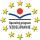In class 6.A are 26 students, and the teacher had managed to give tests to 13 students. 13 testes he gave in 6.5 min. How many pupils still haven't test? How long will it take the teacher to give tests to 43 students?
• A cook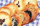A cook wants to make enough cookies for 159 students. The cookie recipe makes 1 1/4 dozen cookies. A dozen equals 12. How many batches does the cook need to make if each student gets one cookie?
• DivisionWhich number in division 16 give 12 and the rest 3?
• SixteenSixteen minus the quotient of 12 and what number is thirteen?
• Each year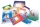Each year, Mr. Baumann purchases enough paper for his 16 students. If each student will use 3/4 pack of paper, how many packs of paper will he need to purchase? Answer as a mixed number or as a whole number.
• Ms. DiSalvatore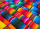Ms. DiSalvatore has 31 ½ feet of ribbon that she is going to use for a class project. Each student needs to have 1 ¾ feet of ribbon. How many students will she be able to give an equal amount of ribbon to?
• Exhibition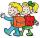The teacher paid 280 Kč for 4.A students for admission to the exhibition. How many students were at the exhibition?
• English and math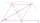1/5 of a class study English, and 3/5 study mathematics. If the class consist of 80 students, how many students study English and mathematics in the class?
• How many 16How many three-tenths are there in two and one-fourths?
• DivisibilityDetermine the smallest integer which divided 11 gives remainder 4 when divided 15 gives remainder 10 and when divided by 19 gives remainder 16.
• Complicated sum minus productWhat must be subtracted from the sum of 3/8 and 5/16 to get difference equal to the product of 5/8 and 3/16?
• ShirtsIn a classroom, 1/6 of the students are wearing blue shirts and 2/3 are wearing white shirts. There are 36 students in the class. How many students are wearing a shirt other than blue or white?
• DozenWhat is the product of 26 and 5? Write the answer in an Arabic numeral. Add up the digits. How many of this is in a dozen? Divide #114 by this
• Class 8.AThree quarters of class 8.A went skiing. Of those who remained at home one third was ill and the remaining six were on math olympic. How many students have class 8.A?
• Evaluate 12Evaluate and simplify 9 divided by 7/6.
• Sayavong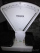Sayavong is making cookies for the class. He has a recipe that calls for 3 and 1/2 cups of flour. He has 7/8 of a cup of wheat flour, and 2 and 1/2 cups of white flour. Does Mr. Sayavong have enough flour to make the cookies?